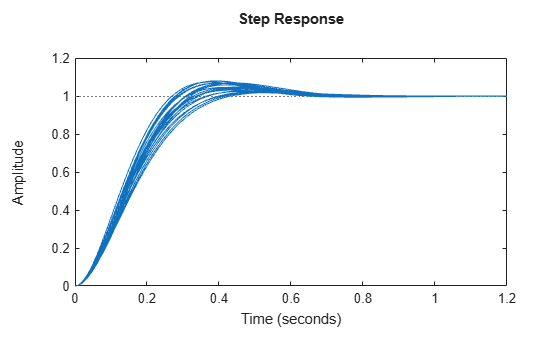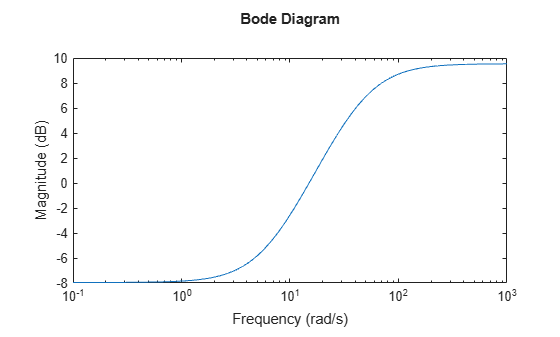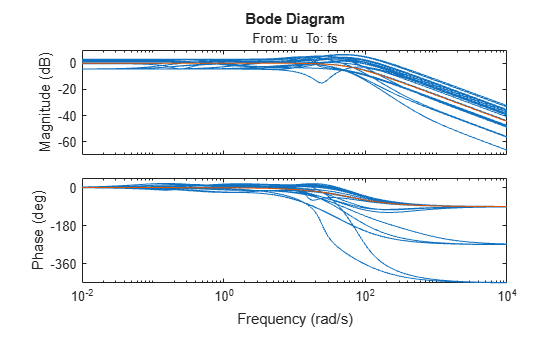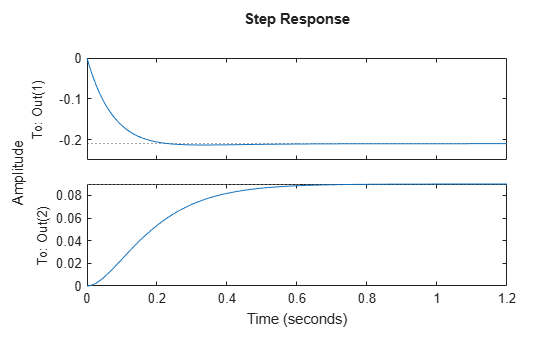# uss

Uncertain state-space model

## Description

Use `uss` model objects to represent uncertain dynamic systems.

The two dominant forms of model uncertainty are:

• Uncertainty in parameters of the underlying differential equation models (uncertain state-space matrices)

• Frequency-domain uncertainty, which often quantifies model uncertainty by describing absolute or relative uncertainty in the frequency response (uncertain or unmodeled linear dynamics)

`uss` model objects can represent dynamic systems with either or both forms of uncertainty. You can use `uss` to perform robust stability and performance analysis and to test the robustness of controller designs.

## Creation

There are several ways to create a `uss` model object, including:

• Use `tf` with one or more uncertain real parameters (`ureal`). For example:

```p = ureal('p',1); usys = tf(p,[1 p]);```

For another example, see Transfer Function with Uncertain Coefficients.

• Use `ss` with uncertain state-space matrices (`umat`). For example:

```p = ureal('p',1); A = [0 3*p; -p p^2]; B = [0; p]; C = ones(2); D = zeros(2,1); usys = ss(A,B,C,D);```

For another example, see Uncertain State-Space Model.

• Combine numeric LTI models with uncertain elements using model interconnection commands such as `connect`, `series`, or `parallel`, or model arithmetic operators such as *, +, or -. For example:

``` sys = tf(1,[1 1]); p = ureal('p',1); D = ultidyn('Delta',[1 1]); usys = p*sys*(1 + 0.1*D);```

For another example, see System with Uncertain Dynamics.

• Convert a double array or a numeric LTI model to `uss` form using `usys = uss(sys)`. In this case, the resulting `uss` model object has no uncertain elements. For example:

```M = tf(1,[1 1 1]); usys = uss(M);```
• Use `ucover` to create a `uss` model whose range of possible frequency responses includes all responses in an array of numeric LTI models. The resulting model expresses the range of behaviors as dynamic uncertainty (`ultidyn`).

## Properties

expand all

Nominal value of the uncertain model, specified as a state-space (`ss`) model object. The state-space model is obtained by setting all the uncertain control design blocks of the uncertain model to their nominal values.

Uncertain elements of the model, specified as a structure whose fields are the names of the uncertain blocks, and whose values are the control design blocks themselves. Thus, the values stored in the structure can be `ureal`, `umat`, `ultidyn`, or other uncertain control design blocks. For instance, the following commands create an uncertain model `usys` with two uncertain parameters, `p1` and `p2`.

```p1 = ureal('p1',1); p2 = ureal('p2',3); A = [0 3*p1; -p1 p1^2]; B = [0; p2]; C = ones(2); D = zeros(2,1); usys = ss(A,B,C,D);```

The `Uncertainty` property of `usys` is a structure with two fields, `p1` and `p2`, whose values are the corresponding `ureal` uncertain parameters.

`usys.Uncertainty`
```ans = struct with fields: p1: [1×1 ureal] p2: [1×1 ureal] ```

You can access or examine each uncertain parameter individually. For example:

`get(usys.Uncertainty.p1)`
``` NominalValue: 1 Mode: 'PlusMinus' Range: [0 2] PlusMinus: [-1 1] Percentage: [-100 100] AutoSimplify: 'basic' Name: 'p1'```

State-space matrices, specified as numeric matrices or uncertain matrices (`umat`). The state-space matrices are evaluated by fixing all dynamic uncertainty blocks (`udyn`, `ultidyn`) to their nominal values.

• `A` — State matrix A, specified as a square matrix or `umat` with as many rows and columns as there are system states.

• `B` — Input-to-state matrix B, specified as a matrix or `umat` with as many rows as there are system states and as many columns as there are system inputs.

• `C` — State-to-output matrix C, specified as a matrix or `umat` with as many rows as there are system outputs and as many columns as there are system states.

• `D` — Feedthrough matrix D, specified as a matrix or `umat` with as many rows as there are system outputs and as many columns as there are system inputs.

• `E`E matrix for implicit (descriptor) state-space models, specified as a matrix or `umat` of the same dimensions as `A`. By default `E = []`, meaning that the state equation is explicit. To specify an implicit state equation E dx/dt = Ax + Bu, set this property to a square matrix of the same size as `A`. See `dss` for more information about descriptor state-space models.

State names, specified as one of these values:

• Character vector — For first-order models

• Cell array of character vectors — For models with two or more states

• `''` — For unnamed states

You can specify `StateName` using a string, such as `"velocity"`, but the state name is stored as a character vector, `'velocity'`.

Example: `'velocity'`

Example: `{'x1','x2'}`

State units, specified as one of these values:

• Character vector — For first-order models

• Cell array of character vectors — For models with two or more states

• `''` — For states without specified units

Use `StateUnit` to keep track of the units each state is expressed in. `StateUnit` has no effect on system behavior.

You can specify `StateUnit` using a string, such as `"mph"`, but the state units are stored as a character vector, `'mph'`.

Example: `'mph'`

Example: `{'rpm','rad/s'}`

Internal delays, specified as a scalar or vector. For continuous-time models, internal delays are expressed in the time unit specified by the `TimeUnit` property of the model object. For discrete-time models, internal delays are expressed as integer multiples of the sample time `Ts`. For example, `InternalDelay = 3` means a delay of three sampling periods.

You can modify the values of internal delays. However, the number of entries in `InternalDelay` cannot change, because it is a structural property of the model.

Internal delays arise, for example, when closing feedback loops on systems with delays, or when connecting delayed systems in series or parallel. For more information about internal delays, see Closing Feedback Loops with Time Delays.

Delay at each input, specified as a scalar or a vector. For a system with `Nu` inputs, set `InputDelay` to an `Nu`-by-1 vector. Each entry of this vector is a numerical value that represents the input delay for the corresponding input channel. For continuous-time models, specify input delays in the time unit stored in the `TimeUnit` property of the model object. For discrete-time models, specify input delays in integer multiples of the sample time `Ts`. For example, `InputDelay = 3` means a delay of three sample times.

Set `InputDelay` to a scalar value to apply the same delay to all channels.

Delay at each output, specified as a scalar or a vector. For a system with `Ny` outputs, set `OutputDelay` to an `Ny`-by-1 vector. Each entry of this vector is a numerical value that represents the output delay for the corresponding output channel. For continuous-time models, specify output delays in the time unit stored in the `TimeUnit` property of the model object. For discrete-time models, specify output delays in integer multiples of the sample time `Ts`. For example, `OutputDelay = 3` means a delay of three sample times.

Set `OutputDelay` to a scalar value to apply the same delay to all channels.

Sample time, specified as:

• 0 — For continuous-time models.

• Positive scalar value — For discrete-time models. Specify the sample time in the units given in the `TimeUnit` property of the model.

• –1 — For discrete-time models with unspecified sample time.

Changing this property does not discretize or resample the model.

Model time units, specified as one of these values:

• `'nanoseconds'`

• `'microseconds'`

• `'milliseconds'`

• `'seconds'`

• `'minutes'`

• `'hours'`

• `'days'`

• `'weeks'`

• `'months'`

• `'years'`

You can specify `TimeUnit` using a string, such as `"hours"`, but the time units are stored as a character vector, `'hours'`.

Model properties such as sample time `Ts`, `InputDelay`, `OutputDelay`, and other time delays are expressed in the units specified by `TimeUnit`. Changing this property has no effect on other properties, and therefore changes the overall system behavior. Use to convert between time units without modifying system behavior.

Names of input channels, specified as one of these values:

• Character vector — For single-input models

• Cell array of character vectors — For models with two or more inputs

• `''` — For inputs without specified names

You can use automatic vector expansion to assign input names for multi-input models. For example, if `sys` is a two-input model, enter:

`sys.InputName = 'controls';`

The input names automatically expand to `{'controls(1)';'controls(2)'}`.

You can use the shorthand notation `u` to refer to the `InputName` property. For example, `sys.u` is equivalent to `sys.InputName`.

Input channel names have several uses, including:

• Identifying channels on model display and plots

• Extracting subsystems of MIMO systems

• Specifying connection points when interconnecting models

You can specify `InputName` using a string, such as `"voltage"`, but the input name is stored as a character vector, `'voltage'`.

Units of input signals, specified as one of these values:

• Character vector — For single-input models

• Cell array of character vectors — For models with two or more inputs

• `''` — For inputs without specified units

Use `InputUnit` to keep track of the units each input signal is expressed in. `InputUnit` has no effect on system behavior.

You can specify `InputUnit` using a string, such as `"voltage"`, but the input units are stored as a character vector, `'voltage'`.

Example: `'voltage'`

Example: `{'voltage','rpm'}`

Input channel groups, specified as a structure where the fields are the group names and the values are the indices of the input channels belonging to the corresponding group. When you use `InputGroup` to assign the input channels of MIMO systems to groups, you can refer to each group by name when you need to access it. For example, suppose you have a five-input model `sys`, where the first three inputs are control inputs and the remaining two inputs represent noise. Assign the control and noise inputs of `sys` to separate groups.

```sys.InputGroup.controls = [1:3]; sys.InputGroup.noise = [4 5];```

Use the group name to extract the subsystem from the control inputs to all outputs.

`sys(:,'controls')`

Example: `struct('controls',[1:3],'noise',[4 5])`

Names of output channels, specified as one of these values:

• Character vector — For single-output models

• Cell array of character vectors — For models with two or more outputs

• `''` — For outputs without specified names

You can use automatic vector expansion to assign output names for multi-output models. For example, if `sys` is a two-output model, enter:

`sys.OutputName = 'measurements';`

The output names automatically expand to `{'measurements(1)';'measurements(2)'}`.

You can use the shorthand notation `y` to refer to the `OutputName` property. For example, `sys.y` is equivalent to `sys.OutputName`.

Output channel names have several uses, including:

• Identifying channels on model display and plots

• Extracting subsystems of MIMO systems

• Specifying connection points when interconnecting models

You can specify `OutputName` using a string, such as `"rpm"`, but the output name is stored as a character vector, `'rpm'`.

Units of output signals, specified as one of these values:

• Character vector — For single-output models

• Cell array of character vectors — For models with two or more outputs

• `''` — For outputs without specified units

Use `OutputUnit` to keep track of the units each output signal is expressed in. `OutputUnit` has no effect on system behavior.

You can specify `OutputUnit` using a string, such as `"voltage"`, but the output units are stored as a character vector, `'voltage'`.

Example: `'voltage'`

Example: `{'voltage','rpm'}`

Output channel groups, specified as a structure where the fields are the group names and the values are the indices of the output channels belonging to the corresponding group. When you use `OutputGroup` to assign the output channels of MIMO systems to groups, you can refer to each group by name when you need to access it. For example, suppose you have a four-output model `sys`, where the second output is a temperature, and the rest are state measurements. Assign these outputs to separate groups.

```sys.OutputGroup.temperature = ; sys.OutputGroup.measurements = [1 3 4];```

Use the group name to extract the subsystem from all inputs to the measurement outputs.

`sys('measurements',:)`

Example: `struct('temperature',,'measurement',[1 3 4])`

Text notes about the model, stored as a string or a cell array of character vectors. The property stores whichever of these two data types you provide. For instance, suppose that `sys1` and `sys2` are dynamic system models, and set their `Notes` properties to a string and a character vector, respectively.

```sys1.Notes = "sys1 has a string."; sys2.Notes = 'sys2 has a character vector.'; sys1.Notes sys2.Notes```
```ans = "sys1 has a string." ans = 'sys2 has a character vector.' ```

Data of any kind that you want to associate and store with the model, specified as any MATLAB® data type.

Model name, stored as a character vector. You can specify `Name` using a string, such as `"DCmotor"`, but the output units are stored as a character vector, `'DCmotor'`.

Example: `'system_1'`

Sampling grid for model arrays, specified as a structure. For model arrays that are derived by sampling one or more independent variables, this property tracks the variable values associated with each model in the array. This information appears when you display or plot the model array. Use this information to trace results back to the independent variables.

Set the field names of the data structure to the names of the sampling variables. Set the field values to the sampled variable values associated with each model in the array. All sampling variables should be numeric and scalar valued, and all arrays of sampled values should match the dimensions of the model array.

For example, suppose you create a 11-by-1 array of linear models, `sysarr`, by taking snapshots of a linear time-varying system at times `t = 0:10`. The following code stores the time samples with the linear models.

` sysarr.SamplingGrid = struct('time',0:10)`

Similarly, suppose you create a 6-by-9 model array, `M`, by independently sampling two variables, `zeta` and `w`. The following code attaches the `(zeta,w)` values to `M`.

```[zeta,w] = ndgrid(<6 values of zeta>,<9 values of w>) M.SamplingGrid = struct('zeta',zeta,'w',w)```

When you display `M`, each entry in the array includes the corresponding `zeta` and `w` values.

`M`
```M(:,:,1,1) [zeta=0.3, w=5] = 25 -------------- s^2 + 3 s + 25 M(:,:,2,1) [zeta=0.35, w=5] = 25 ---------------- s^2 + 3.5 s + 25 ...```

For model arrays generated by linearizing a Simulink® model at multiple parameter values or operating points, the software populates `SamplingGrid` automatically with the variable values that correspond to each entry in the array. For example, the Simulink Control Design™ commands `linearize` (Simulink Control Design) and `slLinearizer` (Simulink Control Design) populate `SamplingGrid` in this way.

## Object Functions

Most functions that work on numeric LTI models also work on `uss` models. These include model interconnection functions such as `connect` and `feedback`, and linear analysis functions such as `bode` and `stepinfo`. Some functions that generate plots, such as `bode` and `step`, plot random samples of the uncertain model to give you a sense of the distribution of uncertain dynamics. When you use these commands to return data, however, they operate on the nominal value of the system only.

In addition, you can use functions such as `robstab` and `wcgain` to perform robustness and worst-case analysis of uncertain systems represented by `uss` models. You can also use tuning functions such as `systune` for robust controller tuning.

The following lists contain a representative subset of the functions you can use with `uss` models.

expand all

 `feedback` Feedback connection of multiple models `connect` Block diagram interconnections of dynamic systems `series` Series connection of two models `parallel` Parallel connection of two models
 `step` Step response plot of dynamic system; step response data `bode` Bode plot of frequency response, or magnitude and phase data `sigma` Singular value plot of dynamic system `margin` Gain margin, phase margin, and crossover frequencies `diskmargin` Disk-based stability margins of feedback loops
 `usample` Generate random samples of uncertain model or element `robstab` Robust stability of uncertain system `robgain` Robust performance of uncertain system `wcgain` Worst-case gain of uncertain system `wcsigmaplot` Plot worst-case gain of uncertain system
 `musyn` Robust controller design using mu synthesis `systune` Tune fixed-structure control systems modeled in MATLAB

## Examples

collapse all

Create a second-order transfer function with uncertain natural frequency and damping coefficient.

```w0 = ureal('w0',10); zeta = ureal('zeta',0.7,'Range',[0.6,0.8]); usys = tf(w0^2,[1 2*zeta*w0 w0^2])```
```Uncertain continuous-time state-space model with 1 outputs, 1 inputs, 2 states. The model uncertainty consists of the following blocks: w0: Uncertain real, nominal = 10, variability = [-1,1], 5 occurrences zeta: Uncertain real, nominal = 0.7, range = [0.6,0.8], 1 occurrences Type "usys.NominalValue" to see the nominal value and "usys.Uncertainty" to interact with the uncertain elements. ```

`usys` is an uncertain state-space (`uss`) model with two Control Design Blocks. The uncertain real parameter `w0` occurs five times in the transfer function, twice in the numerator and three times in the denominator. To reduce the number of occurrences, you can rewrite the transfer function by dividing numerator and denominator by `w0^2`.

`usys = tf(1,[1/w0^2 2*zeta/w0 1])`
```Uncertain continuous-time state-space model with 1 outputs, 1 inputs, 2 states. The model uncertainty consists of the following blocks: w0: Uncertain real, nominal = 10, variability = [-1,1], 3 occurrences zeta: Uncertain real, nominal = 0.7, range = [0.6,0.8], 1 occurrences Type "usys.NominalValue" to see the nominal value and "usys.Uncertainty" to interact with the uncertain elements. ```

In the new formulation, there are only three occurrences of the uncertain parameter `w0`. Reducing the number of occurrences of a Control Design Block in a model can improve the performance of calculations involving the model.

Examine the step response of the system to get a sense of the range of responses that the uncertainty represents.

`step(usys)`When you use linear analysis commands like `step` and `bode` to create response plots of uncertain systems, they automatically plot random samples of the system. While these samples give you a sense of the range of responses that fall within the uncertainty, they do not necessarily include the worst-case response. To analyze worst-case responses of uncertain systems, use `wcgain` or `wcsigmaplot`.

To create an uncertain state-space model, you first use Control Design Blocks to create uncertain elements. Then, use the elements to specify the state-space matrices of the system.

For instance, create three uncertain real parameters and build state-spaces matrices from them.

```p1 = ureal('p1',10,'Percentage',50); p2 = ureal('p2',3,'PlusMinus',[-.5 1.2]); p3 = ureal('p3',0); A = [-p1 p2; 0 -p1]; B = [-p2; p2+p3]; C = [1 0; 1 1-p3]; D = [0; 0];```

The matrices constructed with uncertain parameters, `A`, `B`, and `C`, are uncertain matrix (`umat`) objects. Using them as inputs to `ss` results in a 2-output, 1-input, 2-state uncertain system.

`sys = ss(A,B,C,D)`
```Uncertain continuous-time state-space model with 2 outputs, 1 inputs, 2 states. The model uncertainty consists of the following blocks: p1: Uncertain real, nominal = 10, variability = [-50,50]%, 2 occurrences p2: Uncertain real, nominal = 3, variability = [-0.5,1.2], 2 occurrences p3: Uncertain real, nominal = 0, variability = [-1,1], 2 occurrences Type "sys.NominalValue" to see the nominal value and "sys.Uncertainty" to interact with the uncertain elements. ```

The display shows that the system includes the three uncertain parameters.

Create an uncertain system comprising a nominal model with a frequency-dependent amount of uncertainty. You can model such uncertainty using `ultidyn` and a weighting function that represents the frequency profile of the uncertainty. Suppose that at low frequency, below 3 rad/s, the model can vary up to 40% from its nominal value. Around 3 rad/s, the percentage variation starts to increase. The uncertainty crosses 100% at 15 rad/s and reaches 2000% at approximately 1000 rad/s. Create a transfer function with an appropriate frequency profile, `Wunc`, to use as a weighting function that modulates the amount of uncertainty with frequency.

```Wunc = makeweight(0.40,15,3); bodemag(Wunc)```Next, create a transfer function representing the nominal value of the system. For this example, use a transfer function with a single pole at s = –60 rad/s. Then, create a `ultidyn` model to represent 1-input, 1-output uncertain dynamics, and add the weighted uncertainty to the nominal transfer function.

```sysNom = tf(1,[1/60 1]); unc = ultidyn('unc',[1 1],'SampleStateDim',3); % samples of uncertain dynamics have three states usys = sysNom*(1 + Wunc*unc); % Set properties of usys usys.InputName = 'u'; usys.OutputName = 'fs';```

Examine random samples of usys to see the effect of the uncertain dynamics.

`bode(usys,usys.Nominal)``uss` models, like all model objects, include properties that store dynamics and model metadata. View the properties of an uncertain state-space model.

```p1 = ureal('p1',10,'Percentage',50); p2 = ureal('p2',3,'PlusMinus',[-.5 1.2]); p3 = ureal('p3',0); A = [-p1 p2; 0 -p1]; B = [-p2; p2+p3]; C = [1 0; 1 1-p3]; D = [0; 0]; sys = ss(A,B,C,D); % create uss model get(sys)```
``` NominalValue: [2x1 ss] Uncertainty: [1x1 struct] A: [2x2 umat] B: [2x1 umat] C: [2x2 umat] D: [2x1 double] E: [] StateName: {2x1 cell} StateUnit: {2x1 cell} InternalDelay: [0x1 double] InputDelay: 0 OutputDelay: [2x1 double] InputName: {''} InputUnit: {''} InputGroup: [1x1 struct] OutputName: {2x1 cell} OutputUnit: {2x1 cell} OutputGroup: [1x1 struct] Notes: [0x1 string] UserData: [] Name: '' Ts: 0 TimeUnit: 'seconds' SamplingGrid: [1x1 struct] ```

Most of the properties behave similarly to how they behave for `ss` model objects. The `NominalValue` property is itself an `ss` model object. You can therefore analyze the nominal value as you would any state-space model. For instance, compute the poles and step response of the nominal system.

`pole(sys.NominalValue)`
```ans = 2×1 -10 -10 ```
`step(sys.NominalValue)`As with the uncertain matrices (`umat`), the `Uncertainty` property is a structure containing the uncertain elements. You can use this property for direct access to the uncertain elements. For instance, check the `Range` of the uncertain element named `p2` within `sys`.

`sys.Uncertainty.p2.Range`
```ans = 1×2 2.5000 4.2000 ```

Change the uncertainty range of `p2` within `sys`.

`sys.Uncertainty.p2.Range = [2 4];`

This command changes only the range of the parameter called `p2` in `sys`. It does not change the variable `p2` in the MATLAB workspace.

`p2.Range`
```ans = 1×2 2.5000 4.2000 ```

## Version History

Introduced before R2006a